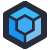Russell F sends us this C# "fuction", and I have to be honest: I have no idea what it's supposed to do. I can trace through the logic, I can see what it does, but I don't understand why it does it.

``````private List<LaborService> Tranpose(List<LaborService> laborService)
{
int half = (int)Math.Ceiling((decimal)(laborService.Count)/2);
for (int i = 0; i < laborService.Count; i++)
{
if (i < half)
laborService[i].Order = 2 * i;
else
laborService[i].Order = (i - half) + 1;
}
return laborService.OrderBy(x => x.Order).ToList();
}
``````

So this starts by finding the rough midpoint of our list. Then we iterate across each element, and if it's position is less than half, we place double its index into the `Order` field. If it's half or greater, we store its index minus half, plus one, into its order field. Finally, we sort by `Order`.

Now, based on the name, we can assume this was inspired by a matrix transposition- oh, I'm sorry, tranposition- based on the method name. It isn't one. It's almost an interleaving operation, but it also isn't one of those.

You can play with the code or just look at this table.

``````Ceiling of half of 10 is 5.
Indexes:  0 1 2 3 4 5 6 7 8 9
Values:   A B C D E F G H I J
Order:    0 2 4 6 8 1 2 3 4 5
-----------------------------
New Sort: A F B G H C I J D E
``````

What you can notice here is that as we re-number our `Order`s, the bottom half gets doubled, but the top half increases incrementally. This means that we end up with ties, and that means that we end up with sections where elements from the either half of the list end up next to each other- see `G, H`, `I,J` and `D, E` in my example.

What is this for? Why does this exist? Why does it matter? No idea.

But Russell has another detail to add:

The Order field is never used anywhere but in this one function -- it appears to have been added solely to allow this.[Advertisement] Keep the plebs out of prod. Restrict NuGet feed privileges with ProGet. Learn more.